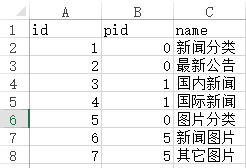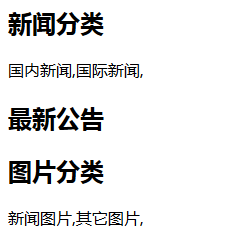详解PHP中foreach的用法和实例

foreach有两种语法：

1、我们用第一种foreach方法来输出。

 1 2 3 foreach ($a as $value) {   echo $value." "; } 最后得到的结果是： Tom Mary Peter Jack 2、我们用第二种 foreach 方法来输出。  1 2 3 foreach ($a as $key => $value) {   echo $key.','.$value."
"; }

0,Tom
1,Mary
2,Peter
3,Jack

 1 $b = array('a'=>'Tom','b'=>'Mary','c'=>'Peter','d'=>'Jack'); 也有人爱这么写，如下：  1 2 3 4 5 6 $b = array(   'a'=>'Tom',   'b'=>'Mary',   'c'=>'Peter',   'd'=>'Jack' );

1、我们用第一种foreach方法来输出和上边一样。

 1 2 3 foreach ($b as $value) {   echo $value." "; } 最后得到的结果是： Tom Mary Peter Jack 2、我们用第二种foreach方法来输出。  1 2 3 foreach ($b as $key => $value) {   echo $key.','.$value."
"; }

a,Tom
b,Mary
c,Peter
d,Jack

1、我们采用第一种 foreach方法：

 1 2 3 4 foreach ($c as $value) {   print_r($value);  echo " "; } 得到这样的结果： Array (  => 11  => Tom ) Array (  => 22  => Mary ) Array (  => 33  => Peter ) Array (  => 44  => Jack ) 2、我们采用第二种foreach方法：  1 2 3 4 5 foreach ($c as $key => $value) {   echo '$key='.$key."
";   print_r($value);  echo " "; } 得到如下的结果:$key=0
Array (  => 11  => Tom )
$key=1 Array (  => 22  => Mary )$key=2
Array (  => 33  => Peter )
$key=3 Array (  => 44  => Jack ) 总结：由上可知，基本二维数组，$key就是序号 ,如 0\1\2\3 等等！

 1 2 3 4 5 6 $d = array(  array('id'=>'11','name'=>'Tom'),  array('id'=>'22','name'=>'Mary'),  array('id'=>'33','name'=>'Peter'),  array('id'=>'44','name'=>'Jack') ); 1、用第一种方法代码：  1 2 3 4 foreach ($d as $value) {  print_r($value);   echo "
"; }

Array ( [id] => 11 [name] => Tom )
Array ( [id] => 22 [name] => Mary )
Array ( [id] => 33 [name] => Peter )
Array ( [id] => 44 [name] => Jack )

2、用第二种方法的代码：

 1 2 3 4 5 foreach ($d as $key => $value) {  echo '$key='.$key." ";  print_r($value);   echo "
"; }

$key=0 Array ( [id] => 11 [name] => Tom )$key=1
Array ( [id] => 22 [name] => Mary )
$key=2 Array ( [id] => 33 [name] => Peter )$key=3
Array ( [id] => 44 [name] => Jack )

 1 2 3 4 foreach ($d as $key => $value) {  echo ($value['name']);   echo "
"; }

 1 2 3 4 5 6 //获取name列作为一维数组 $nameArr = array(); //name列 foreach ($d as $key => $value) {   $nameArr[] = $value['name']; } print_r($nameArr); 以上通过赋空数组值，foreach 这个空数组等于我们的值，就得到了一个新的数组！以上代码的结果如下：  1 2 3 4 5 6 7 Array (   => Tom   => Mary   => Peter   => Jack ) 这个数组明显是：一维普通数组，如下：  1 $d = array('Tom','Mary','Peter','Jack');

 1 2 3 4 5 6 7 8 9 10 //从数据库中取出的分类数据 $original_array = array(  array('id' => 1,'pid' => 0,'name' => '新闻分类'),  array('id' => 2,'pid' => 0,'name' => '最新公告'),  array('id' => 3,'pid' => 1,'name' => '国内新闻'),  array('id' => 4,'pid' => 1,'name' => '国际新闻'),  array('id' => 5,'pid' => 0,'name' => '图片分类'),  array('id' => 6,'pid' => 5,'name' => '新闻图片'),  array('id' => 7,'pid' => 5,'name' => '其它图片') ); 同时，数据库是这个样子的。说明：数据库的分类就是这个样子的！取出来的数组也是这个样子的！一般这样子的！  1 2 3 4 5 6 7 8 9 10 11 12 13 14 15 16 17 18 19 20 21 22 23 24 25 26 27 28 29 30 31 32 33 34 35 36 37 38 //从数据库中取出的分类数据 $original_array = array(   array(     'id' => 1,     'pid' => 0,     'name' => '新闻分类'   ),   array(     'id' => 2,     'pid' => 0,     'name' => '最新公告'   ),   array(     'id' => 3,     'pid' => 1,     'name' => '国内新闻'   ),   array(     'id' => 4,     'pid' => 1,     'name' => '国际新闻'   ),   array(     'id' => 5,     'pid' => 0,     'name' => '图片分类'   ),   array(     'id' => 6,     'pid' => 5,     'name' => '新闻图片'   ),   array(     'id' => 7,     'pid' => 5,     'name' => '其它图片'   ) );

 1 2 3 4 5 6 7 8 9 10 11 12 13 14 15 16 17 18 19 20 21 22 23 24 25 26 27 28 29 30 31 32 33 34 35 36 37 38 39 40 41 42 //整理后的分类数据 $output_array = array(  array(  'id' => 1,  'pid' => 0,  'name' => '新闻分类',  'children' => array(  array(  'id' => 3,  'pid' => 1,  'name' => '国内新闻'  ),  array(  'id' => 4,  'pid' => 1,  'name' => '国际新闻'  ),  ),  ),  array(  'id' => 2,  'pid' => 0,  'name' => '最新公告',  ),  array(  'id' => 5,  'pid' => 0,  'name' => '图片分类',  'children' => array(  array(  'id' => 6,  'pid' => 5,  'name' => '新闻图片'  ),  array(  'id' => 7,  'pid' => 5,  'name' => '其它图片'  ),  ),  ), ); 很明显，这里数组多了一个字段，就是 children！ 那么，怎么 从$original_array 变为 $output_array呢？这里有我一个朋友做的函数，当然也用到 foreach！ 函数如下：  1 2 3 4 5 6 7 8 9 10 11 12 13 14 15 16 17 18 19 20 21 22 //整理函数 /**  * 生成无限级树算法  * @author Baiyu 2014-04-01  * @param array$arr        输入数组  * @param number $pid 根级的pid  * @param string$column_name    列名,id|pid父id的名字|children子数组的键名  * @return array $ret  */ function make_tree($arr, $pid = 0, $column_name = 'id|pid|children') {   list($idname, $pidname, $cldname) = explode('|', $column_name);   $ret = array();  foreach ($arr as $k => $v) {     if ($v [$pidname] == $pid) {  $tmp = $arr [$k];       unset($arr [$k]);       $tmp [$cldname] = make_tree($arr, $v [$idname], $column_name);       $ret [] = $tmp;     }   }   return $ret; } 那们怎么使用呢？  1 2 //整理函数的使用 $output_array = make_tree($original_array); 完整使用方法如下：  1 $output_array =make_tree($arr, 0, 'id|pid|children') 函数之后，我们这样调用就得到了一级分类与二级分类！  1 2 3 4 5 foreach ($output_array as $key => $value) {   echo '

'.$value['name'].' ';  foreach ($value['children'] as $key => $value) {     echo $value['name'].','; } 结果如下：附：$output_array 这个数组，我们使用print_r，就可以得到如下的结果！

 1 2 3 4 5 6 7 8 9 10 11 12 13 14 15 16 17 18 19 20 21 22 23 24 25 26 27 28 29 30 31 32 33 34 35 36 37 38 39 40 41 42 43 44 45 46 47 48 49 50 51 52 53 54 55 56 57 58 59 60 61 62 63 64 65 66 67 68 69 70 71 72 73 74 75 76 77 78 79 80 Array (    => Array     (       [id] => 1       [pid] => 0       [name] => 新闻分类       [children] => Array         (            => Array             (               [id] => 3               [pid] => 1               [name] => 国内新闻               [children] => Array                 (                 )               )              => Array             (               [id] => 4               [pid] => 1               [name] => 国际新闻               [children] => Array                 (                 )               )           )       )      => Array     (       [id] => 2       [pid] => 0       [name] => 最新公告       [children] => Array         (         )       )      => Array     (       [id] => 5       [pid] => 0       [name] => 图片分类       [children] => Array         (            => Array             (               [id] => 6               [pid] => 5               [name] => 新闻图片               [children] => Array                 (                 )               )              => Array             (               [id] => 7               [pid] => 5               [name] => 其它图片               [children] => Array                 (                 )               )           )       )   )